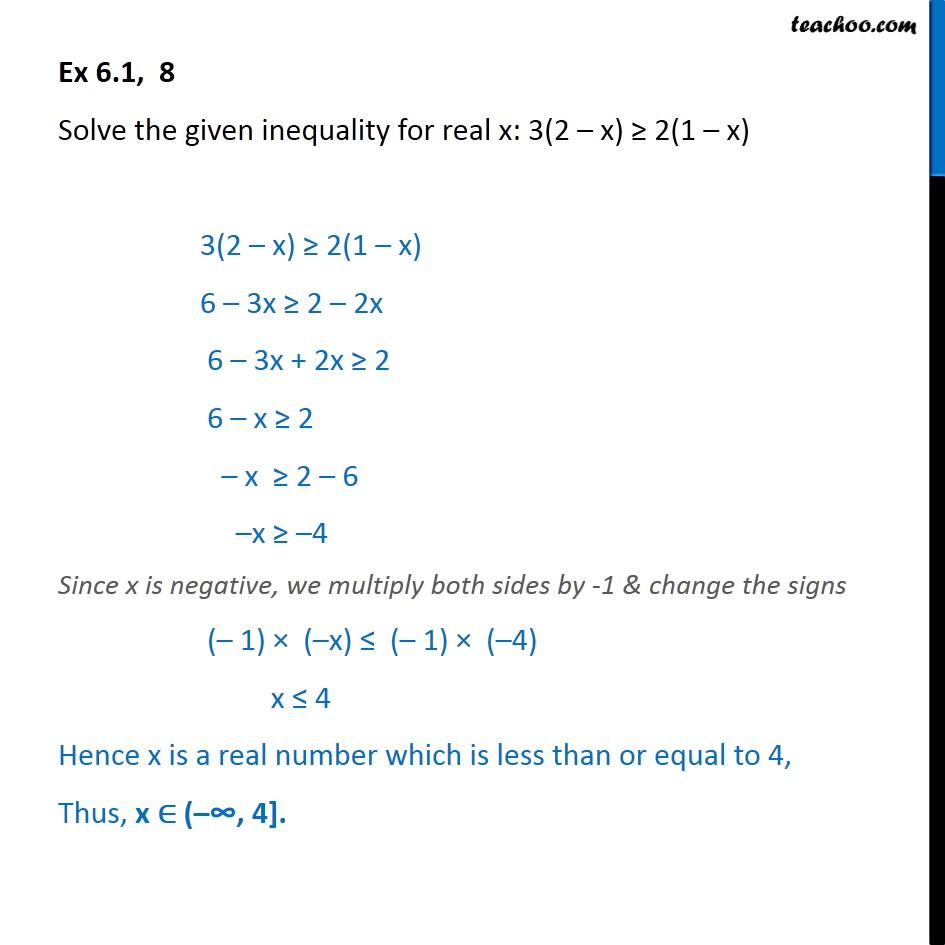Ex 6.1

Chapter 6 Class 11 Linear Inequalities
Serial order wiseGet live Maths 1-on-1 Classs - Class 6 to 12

### Transcript

Ex 6.1, 8 Solve the given inequality for real x: 3(2 – x) ≥ 2(1 – x) 3(2 – x) ≥ 2(1 – x) 6 – 3x ≥ 2 – 2x 6 – 3x + 2x ≥ 2 6 – x ≥ 2 – x ≥ 2 – 6 –x ≥ –4 Since x is negative, we multiply both sides by -1 & change the signs (– 1) × (–x) ≤ (– 1) × (–4) x ≤ 4 Hence x is a real number which is less than or equal to 4, Thus, x ∈ (–𕔴 4].